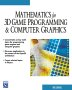# Maths - Combining and Factoring Polynomials

Until now (on this page) we have considered polynomials as being compound types being the sum of terms made up of various powers of one or more variables multiplied by constants. Alternatively one or more variables with any permutation of addition and multiplication.

Here we will consider a polynomial as an element which can be added, subtracted, multiplied and divided just like and other mathematical element like complex numbers or vectors.

Adding and subtracting polynomials is easy, all we do is add or subtract the constants associated with like terms.

Multiplying is just a matter of multiplying out all the terms.

Dividing is more complicated, we can do it in a similar way that we do long division, as with long division we may get a remainder as well as the result.

Factoring, that is finding two or more terms that can be multiplied together to give a specified polynomial, is even more difficult. Over a certain complexity of polynomial the only way might be a trail and error approach.

## Program

There are a number of open source programs that can allow us to work with polynomials. I have used Axiom, how to install Axiom here.

Using this program (user input in red) below we can see how to work with whole polynomials, in this case in two dimensions, we see how to combine polynomials by arithmetic operations and also how the factor them.

In (1) and (2) we define two polynomials 'p1' and 'p2' the program automatically recognises them as polynomials with integer constants.

In (3) to (6) we try adding, subtracting, multiplying and dividing them. In the case of division (6) there is not an exact result so the program leaves the result as a fraction, that is the program does not try to return a result with a remainder. In (7) we do a division that does have an exact result which is returned by the program (Although the type is still given as fraction).

In (8) we show how to factor this polynomial. The result is returned as a 'factored polynomial', that is a number of polynomials multiplied together.

 ```(1) -> p1 := 4*x**3 + 2*y**2 + 1 2 3 (1) 2y + 4x + 1 Type: Polynomial Integer (2) -> p2 := 12*x**5 -x**3*y + 12``` ``` 3 5 (2) - x y + 12x + 12 Type: Polynomial Integer (3) -> p1 + p2``` ``` 2 3 5 3 (3) 2y - x y + 12x + 4x + 13 Type: Polynomial Integer (4) -> p1 - p2``` ``` 2 3 5 3 (4) 2y + x y - 12x + 4x - 11 Type: Polynomial Integer (5) -> p12 := p1 * p2``` ``` 3 3 5 2 6 3 8 5 3 (5) - 2x y + (24x + 24)y + (- 4x - x )y + 48x + 12x + 48x + 12 Type: Polynomial Integer``` `(6) -> p1 / p2` ``` 2 3 - 2y - 4x - 1 (6) --------------- 3 5 x y - 12x - 12 Type: Fraction Polynomial Integer (7) -> p12 / p2``` ``` 2 3 (7) 2y + 4x + 1 Type: Fraction Polynomial Integer (8) -> factor p12``` ``` 3 5 2 3 (8) - (x y - 12x - 12)(2y + 4x + 1) Type: Factored Polynomial Integer```

## Algebraic Numbers

So far, on this page, all the numbers are integers, known as a Z-polynomial, but the general solution to polynomials is of type: AlgebraicNumber. That is complex numbers whose parts are rationals and roots of rationals and all combinations of these with +, -, * and /.

We will denote AlgebraicNumber as Qalg - The root of a Z-polynomial - A complex number made up more than just radical integers although it is closed under sum, difference, product, quotient and nth root. We discuss number theory (types of numbers) on this page.

Since the solutions are of type AlgebraicNumber then we need to factor into parts which have constant terms of this type.

We can take this further on this page we look at polynomial variables and how this is all linked to the mathematics of rings.

 metadata block see also: Correspondence about this page Book Shop - Further reading. Where I can, I have put links to Amazon for books that are relevant to the subject, click on the appropriate country flag to get more details of the book or to buy it from them.Mathematics for 3D game Programming - Includes introduction to Vectors, Matrices, Transforms and Trigonometry. (But no euler angles or quaternions). Also includes ray tracing and some linear & rotational physics also collision detection (but not collision response). Other Math Books Specific to this page here:

This site may have errors. Don't use for critical systems.